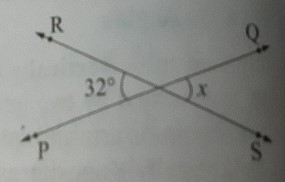"
">

# In the following figure, find the value of x."

Given :

In the given figure, $\angle POR =32°$.

To find :

We have to find the measure of x.

Solution :

$\angle POR$ and x are vertically opposite angles.

We know that vertically opposite angles are equal.

So, $x = 32°$

Therefore, the measure of x is 32°.

Updated on: 10-Oct-2022

32 Views